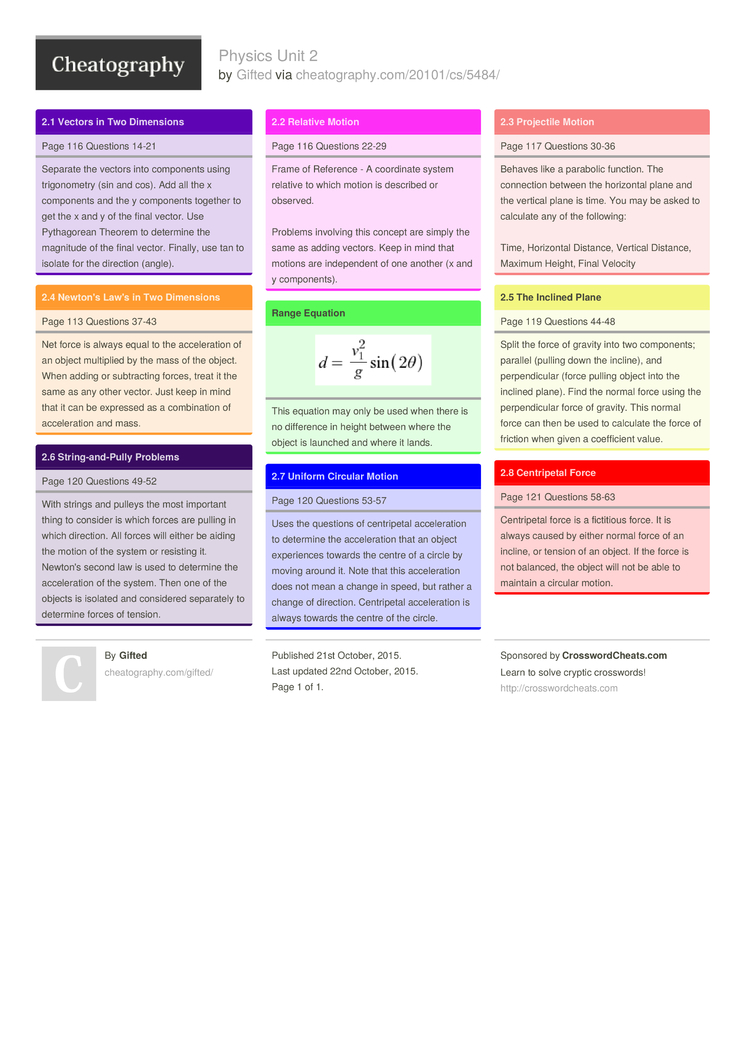# Physics Unit 2 by Gifted

### 2.1 Vectors in Two Dimensions

 Page 116 Questions 14-21
Separate the vectors into components using trigon­ometry (sin and cos). Add all the x components and the y components together to get the x and y of the final vector. Use Pythag­orean Theorem to determine the magnitude of the final vector. Finally, use tan to isolate for the direction (angle).

### 2.4 Newton's Law's in Two Dimensions

 Page 113 Questions 37-43
Net force is always equal to the accele­ration of an object multiplied by the mass of the object. When adding or subtra­cting forces, treat it the same as any other vector. Just keep in mind that it can be expressed as a combin­ation of accele­ration and mass.

### 2.6 String­-an­d-Pully Problems

 Page 120 Questions 49-52
With strings and pulleys the most important thing to consider is which forces are pulling in which direction. All forces will either be aiding the motion of the system or resisting it. Newton's second law is used to determine the accele­ration of the system. Then one of the objects is isolated and considered separately to determine forces of tension.

### 2.2 Relative Motion

 Page 116 Questions 22-29
Frame of Reference - A coordinate system relative to which motion is described or observed.

Problems involving this concept are simply the same as adding vectors. Keep in mind that motions are indepe­ndent of one another (x and y compon­ents).

### Range EquationThis equation may only be used when there is no difference in height between where the object is launched and where it lands.

### 2.7 Uniform Circular Motion

 Page 120 Questions 53-57
Uses the questions of centri­petal accele­ration to determine the accele­ration that an object experi­ences towards the centre of a circle by moving around it. Note that this accele­ration does not mean a change in speed, but rather a change of direction. Centri­petal accele­ration is always towards the centre of the circle.

### 2.3 Projectile Motion

 Page 117 Questions 30-36
Behaves like a parabolic function. The connection between the horizontal plane and the vertical plane is time. You may be asked to calculate any of the following:

Time, Horizontal Distance, Vertical Distance, Maximum Height, Final Velocity

### 2.5 The Inclined Plane

 Page 119 Questions 44-48
Split the force of gravity into two compon­ents; parallel (pulling down the incline), and perpen­dicular (force pulling object into the inclined plane). Find the normal force using the perpen­dicular force of gravity. This normal force can then be used to calculate the force of friction when given a coeffi­cient value.

### 2.8 Centri­petal Force

 Page 121 Questions 58-63
Centri­petal force is a fictitious force. It is always caused by either normal force of an incline, or tension of an object. If the force is not balanced, the object will not be able to maintain a circular motion.1 Page

PDF (recommended)